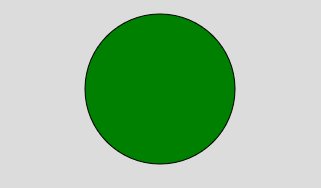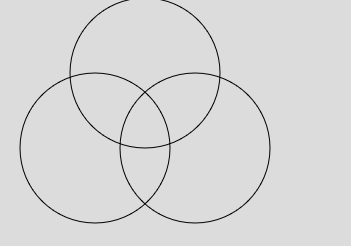GeeksforGeeks App
Open AppBrowser
Continue

## Related Articles

The circle() function is used to draw the circle on the screen. A circle is the closed shape. A circle can be created by using the center and radius of the circle.

Syntax:

`circle(x, y, d)`

Parameters:

• x: It is used to set the x-coordinate of the center of the circle.
• y: It is used to set the y-coordinate of the center of the circle.
• d: It is used to set the diameter of the circle.

Example 1:

 `function` `setup() { ``     ` `    ``// Create Canvas of given size ``    ``createCanvas(400, 300); `` ` `} `` ` `function` `draw() { ``     ` `    ``background(220);``     ` `    ``// Use color() function``    ``let c = color(``'green'``);`` ` `    ``// Use fill() function to fill color``    ``fill(c);``   ` `    ``// Draw a circle ``    ``circle(200, 100, 150); ``   ` `}`

Output:Example 2:

 `function` `setup() { ``     ` `    ``// Create Canvas of given size ``    ``createCanvas(400, 300); `` ` `} `` ` `function` `draw() { ``     ` `    ``background(220);``   ` `    ``// Draw a circle ``    ``circle(200, 150, 150); ``   ` `    ``noFill();``   ` `    ``// Draw a circle ``    ``circle(100, 150, 150); ``   ` `    ``// Draw a circle ``    ``circle(150, 75, 150);``   ` `}`

Output:Reference: https://p5js.org/reference/#/p5/circle

My Personal Notes arrow_drop_up
Related Tutorials